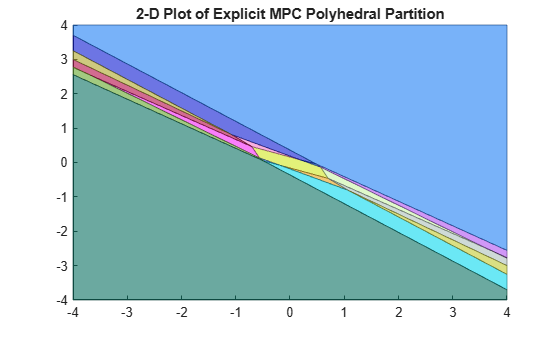# plotSection

Visualize explicit MPC control law as 2-D sectional plot

## Syntax

``plotSection(EMPCobj,plotParams)``

## Description

example

````plotSection(EMPCobj,plotParams)` displays a 2-D sectional plot of the piecewise affine regions used by an explicit MPC controller. All but two of the control law’s free parameters are fixed, as specified by `plotParams`. The two remaining variables form the plot axes. By default, the `EMPCobj.Range` property sets the bounds for these axes. ```

## Examples

collapse all

Define a double integrator plant model and create a traditional implicit MPC controller for this plant. Constrain the manipulated variable to have an absolute value less than `1`.

```plant = tf(1,[1 0 0]); MPCobj = mpc(plant,0.1,10,3);```
```-->The "Weights.ManipulatedVariables" property is empty. Assuming default 0.00000. -->The "Weights.ManipulatedVariablesRate" property is empty. Assuming default 0.10000. -->The "Weights.OutputVariables" property is empty. Assuming default 1.00000. ```
`MPCobj.MV = struct('Min',-1,'Max',1);`

Define the parameter bounds for generating an explicit MPC controller.

`range = generateExplicitRange(MPCobj);`
```-->Converting the "Model.Plant" property to state-space. -->Converting model to discrete time. Assuming no disturbance added to measured output channel #1. -->The "Model.Noise" property is empty. Assuming white noise on each measured output. ```
```range.State.Min(:) = [-10;-10]; range.State.Max(:) = [10;10]; range.Reference.Min(:) = -2; range.Reference.Max(:) = 2; range.ManipulatedVariable.Min(:) = -1.1; range.ManipulatedVariable.Max(:) = 1.1;```

Create an explicit MPC controller.

`EMPCobj = generateExplicitMPC(MPCobj,range);`
```Regions found / unexplored: 19/ 0 ```

Create a default plot parameter structure, which specifies that all of the controller parameters are fixed at their nominal values for plotting.

`plotParams = generatePlotParameters(EMPCobj);`

Allow the controller states to vary when creating a plot.

```plotParams.State.Index = []; plotParams.State.Value = [];```

Fix the manipulated variable and reference signal to `0` for plotting.

```plotParams.ManipulatedVariable.Index(1) = 1; plotParams.ManipulatedVariable.Value(1) = 0; plotParams.Reference.Index(1) = 1; plotParams.Reference.Value(1) = 0;```

Generate the 2-D section plot for the explicit MPC controller.

`plotSection(EMPCobj,plotParams)````ans = Figure (1: PiecewiseAffineSectionPlot) with properties: Number: 1 Name: 'PiecewiseAffineSectionPlot' Color: [1 1 1] Position: [348 480 583 437] Units: 'pixels' Show all properties ```

## Input Arguments

collapse all

Explicit MPC controller for which you want to create a 2-D sectional plot, specified as an Explicit MPC controller object. Use `generateExplicitMPC` to create an explicit MPC controller.

Parameters for sectional plot of explicit MPC control law, specified as a structure. Use `generatePlotParameters` to create an initial structure in which all the parameters of the controller are fixed at their nominal values. Then, modify this structure as necessary before invoking `plotSection`. See `generatePlotParameters` for more information.

## Version History

Introduced in R2014b